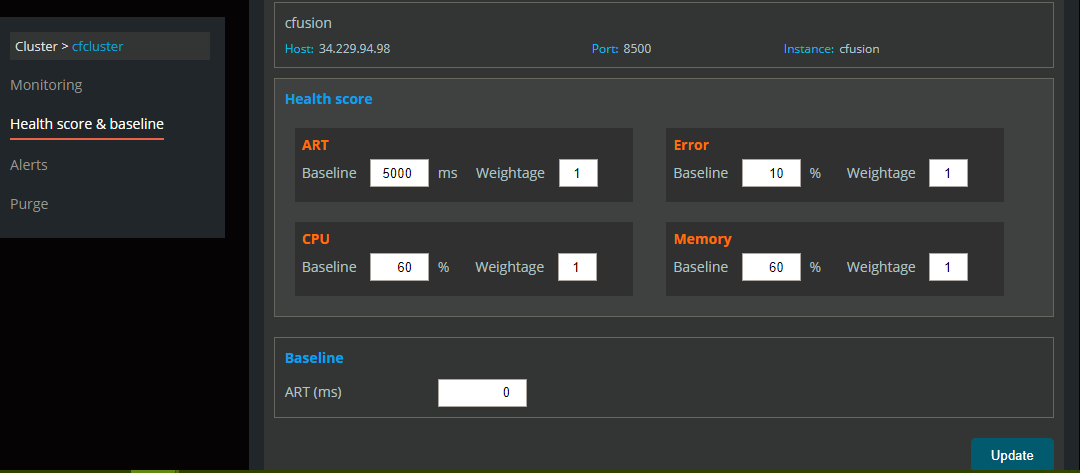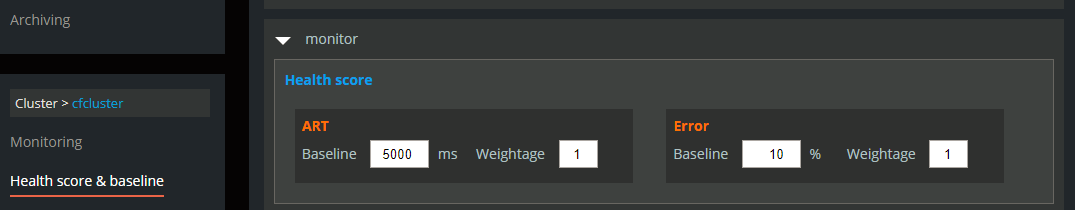The mystery of calculating Health Score in Performance Monitoring Toolset
Performance Monitoring Toolset can measure how your system is performing. In various pages of our brand new toolset, you can find health scores for node/application/cluster/group. These health scores reflect the state of various components in your ColdFusion setup.
We will see how they are calculated and how to configure them.
Node Health Score
Health Score is dependent on 4 parameters. They are – ART, Error rate, CPU usage, and Memory Usage. We calculate scores for each of the 4 parameters as shown below.
• ART Score: To calculate an ART score, the ART of the last 5 minutes is calculated and compared against the ART provided by you.
• For example, if Abaseline  is the baseline ART provided you and Aactual is ART of last 5 minutes and let (2*Abaseline  – Abaseline) / 5 = diff, then,
• ART Score = 100    if Aactual  <=  Abaseline
• ART Score = 83      if Abaseline <= Aactual  <   Abaseline + diff
• ART Score = 66      if Abaseline + diff    <= Aactual  <   Abaseline + 2*diff
• ART Score = 50      if Abaseline + 2*diff<= Aactual  <   Abaseline + 3*diff
• ART Score = 33      if Abaseline + 3*diff<= Aactual  <   Abaseline + 4*diff
• ART Score = 16      if Abaseline + 4*diff<= Aactual  <   Abaseline + 5*diff
• ART Score = 0        if Aactual  >   Abaseline + 5*diff
• Error Score : To calculate Error score, the Error percentage of last 5 minutes is calculated and compared against error percentage provided by the user.
• For example, if Ebaseline  is error baseline provided by user and Eactual is error percentage of last 5 minutes and let (Min(5*Ebaseline  , 100) –  Ebaseline) / 5 = diff, then,
• Error Score = 100    if Eactual  <=  Ebaseline
• Error Score = 83      if Ebaseline <= Eactual  <   Ebaseline + diff
• Error Score = 66      if Ebaseline + diff    <= Eactual  <   Ebaseline + 2*diff
• Errror Score = 50    if Ebaseline + 2*diff<= Eactual  <   Ebaseline + 3*diff
• Error Score = 33      if Ebaseline + 3*diff<= Eactual  <   Ebaseline + 4*diff
• Error Score = 16      if Ebaseline + 4*diff<= Eactual  <   Ebaseline + 5*diff
• Error Score = 0        if Eactual  >  Ebaseline + 5*diff
• CPU Score : To calculate the CPU score, CPU usage percentage of last 5 minutes is calculated and compared against cpu usage percentage provided by the user.
• For example, if Cbaseline  is the CPU usage baseline provided by you and Cactual is the CPU usage percentage of last 5 minutes, and let (Min(5*Cbaseline  , 100) –  Cbaseline) / 5 = diff, then,
• CPU Score = 100    if Cactual  <=  Cbaseline
• CPU Score = 83      if Cbaseline <= Cactual  <   Cbaseline + diff
• CPU Score = 66      if Cbaseline + diff    <= Cactual  <  Cbaseline + 2*diff
• CPU Score = 50      if Cbaseline + 2*diff<= Cactual  <  Cbaseline + 3*diff
• CPU  Score = 33     if Cbaseline + 3*diff<= Cactual  <  Cbaseline + 4*diff
• CPU Score = 16      if Cbaseline + 4*diff<= Cactual  < Cbaseline + 5*diff
• CPU Score = 0        if Cactual  >  Cbaseline + 5*diff
• Memory Score :  To calculate the memory score, the heap usage percentage of the last 5 minutes is calculated and compared against heap usage percentage provided by the user.
• For example, if Mbaseline  is heap usage baseline provided by user and Mactual is heap usage percentage of last 5 minutes and let (Min(5*Mbaseline  , 100) –  Mbaseline) / 5 = diff, then,
• Memory Score = 100    if Mactual  <=  Mbaseline
• Memory Score = 83      if Mbaseline <= Mactual  <   Mbaseline + diff
• Memory Score = 66      if Mbaseline + diff    <= Mactual  <  Mbaseline + 2*diff
• Memory Score = 50      if Mbaseline + 2*diff<= Mactual  <  Mbaseline + 3*diff
• Memory Score = 33       if Mbaseline + 3*diff<= Mactual  <  Mbaseline + 4*diff
• Memory Score = 16      if Mbaseline + 4*diff<= Mactual  < Mbaseline + 5*diff
• Memory Score = 0        if Mactual  >  Mbaseline + 5*diff
• Node Health Score = (ART Score * ART Weightage + Error Score * Error Weightage + CPU Score * CPU Weightage + Memory Score * Memory Weightage) / (ART Weightage + Error Weightage + CPU Weightage + Memory Weightage)

Note that the error score has veto power. This means that if error score is zero, the entire health score becomes zero.

Application Health Score
Health Score is dependent on 2 parameters. They are – ART and Error rate. We calculate scores for each of the 2 parameters as shown below.
• ART Score : To calculate ART score, ART of last 5 minutes is calculated and compared against the ART provided by the user.
• Suppose, Abaseline  is ART baseline provided by user and Aactual is ART of last 5 minutes and let (2*Abaseline  – Abaseline) / 5 = diff
• ART Score = 100    if Aactual  <=  Abaseline
• ART Score = 83      if Abaseline <= Aactual  <   Abaseline + diff
• ART Score = 66      if Abaseline + diff    <= Aactual  <   Abaseline + 2*diff
• ART Score = 50      if Abaseline + 2*diff<= Aactual  <   Abaseline + 3*diff
• ART Score = 33      if Abaseline + 3*diff<= Aactual  <   Abaseline + 4*diff
• ART Score = 16      if Abaseline + 4*diff<= Aactual  <   Abaseline + 5*diff
• ART Score = 0        if Aactual  >  Abaseline + 5*diff
• Error Score : To calculate Error score, Error percentage of last 5 minutes is calculated and compared against error percentage provided by the user.
• Suppose, Ebaseline  is error baseline provided by user and Eactual is error percentage of last 5 minutes and let (Min(5*Ebaseline  , 100) –  Ebaseline) / 5 = diff
• Error Score = 100    if Eactual  <=  Ebaseline
• Error Score = 83      if Ebaseline <= Eactual  <   Ebaseline + diff
• Error Score = 66      if Ebaseline + diff    <= Eactual  <   Ebaseline + 2*diff
• Errror Score = 50    if Ebaseline + 2*diff<= Eactual  <   Ebaseline + 3*diff
• Error Score = 33      if Ebaseline + 3*diff<= Eactual  <   Ebaseline + 4*diff
• Error Score = 16      if Ebaseline + 4*diff<= Eactual  <   Ebaseline + 5*diff
• Error Score = 0        if Eactual  >  Ebaseline + 5*diff
• Application Health Score = (ART Score * ART Weightage + Error Score * Error Weightage) / (ART Weightage + Error Weightage)

Please note that error score has veto power. This means that if error score is zero, entire health score becomes zero.

Cluster Health Score :  Cluster health score is simple mean of all nodes in cluster.
Group Health Score :  Group health score is simple mean of all nodes in group.
Setting Baseline for different parameters:  In Health Score and Baseline section of settings, admin can set baseline and weightage parameters for calculation of various health scores.
For node :For application :